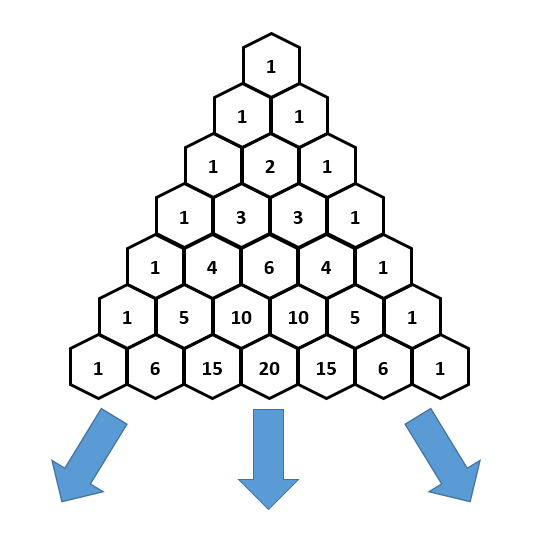Question 1 (Easy)

State whether the following code is safe or not given that \length(A) == 10.

1. 1 == 1 || A
2. 1 == 1 && A
3. 1 == 2 || A
4. 1 == 2 && A

Solutions

1. Safe. 1 == 1 short-circuits the boolean condition.
2. Not safe. Both 1 == 1 and A are checked.
3. Not safe. Since 1 != 2, A is checked.
4. Safe. Since 1 != 2, the condition is short-circuited and is false.

Question 2 (Medium)

Given an integer (32 bits), write a function that extracts the bits and puts them into an array. For example, note that $5_{} = 101_{}$. Then, with an array A having a length of 32, calling int_to_array(5, A) will result in A = [0, 0, ... , 0, 1, 0, 1].

Solution

void int_to_array(int n, int[] A)
//@requires \length(A) == 32;
{
for (int i = 31; i >= 0; i--)
//@loop_invariant 0 <= i && i < 32;
{
// Get the very last bit of n
A[i] = n & 1;

// Shift all the bits of n right by 1
n = n >> 1;
}
}


Question 3 (Easy)

Consider running following code.

int[] A = alloc_array(int, 10);
int[] B;
int[] C;
A = 1;
A = 2;
A = 3;
A = 4;
B = A;
printint(B);
C = A;
printint(C);
printint(C);
C = 7;
B = 3;
printint(C);
printint(C);
C = alloc_array(int, 12);
B = C;
C = 7;
B = 3;
printint(A);
printint(B);


Draw the state of local and allocated memory at the end of this execution. What will be printed in the terminal? How many arrays are there in memory? What are their lengths? Which one is A referring to? What about B and C?

Solution

0123727 will be printed in the terminal. There are two different arrays in memory – one with length 10 and another with length 12. A is pointing to the array with length 10. B and C are both pointing to the same array of length 12.

Question 3 (Medium)

Addressing learning objectives: 1, 2, 4(Image source: Brilliant)

As is shown in the picture above, a Pascal’s triangle is a numerical triangle, where each level stars and ends with $1$, and any other number om the level is the sum of the two numbers above it.

Construct a Pascal’s triangle with nexted arrays int[][] A where A[i] is the $i^\text{th}$ level of the Pascal triangle (the top level of the triangle is level $0$). Note that each level will have a different length.

Solution

int[][] A (int n)
{
alloc_array(int[], n);

for (int i = 0; i < n; i++)
//@loop_invariant 0 <= i && i <= n;
{
A[i] = alloc_array(int, i + 1);

// Set the first and last values to 1
A[i] = 1; A[i][i] = 1;

for (int j = 1; j < i; j++)
//@loop_invariant 1 <= j && j <= i;
{
A[i][j] = A[i - 1][j - 1] + A[i - 1][j];
}
}

return A;


Question 4 (Hard)

Addressing learning objectives: 1, 2, 5

Write a function partial_copy that creates a copy of an array int[] A from index lo to index hi (not including hi). Your function should have the following prototype and satisfies the contracts.

int[] partial_copy(int[] A, int lo, int hi)
//@requires 0 <= lo && lo <= hi && hi <= \length(A)
//@ensures arr_equal(\result, 0, \length(\result), A, lo, hi);


The specification function arr_equal(A1, lo1, hi1, A2, lo2, hi2) returns true if and only if two array segments A1[lo1, hi1) and A2[lo2, hi2) are equal.

Solution

 int[] partial_copy(int[] A, int lo, int hi)
//@requires 0 <= lo && lo <= hi && hi <= \length(A);
//@ensures arr_equal(\result, 0, \length(\result), A, lo, hi);
{
int length = hi - lo;
int[] B = alloc_array(int, length);

for(int a = 0; a < length; a++)
//@loop_invariant 0 <= a && a <= length;
//@loop_invariant arr_equal(B, 0, a, A, lo, lo + a);
{
B[a] = A[lo + a];
}

return B;
}


Proof for postcondition: When the loop terminates, we have

1. length = hi - lo, by line 5
2. a >= length, by line 7
3. a <= length, by line 8
4. a == length, by (2) and (3)
5. arr_equal(B, 0, a, A, lo, lo + a), by line 9
6. arr_equal(B, 0, length, A, lo, lo + length), by (4) and (5)
7. arr_equal(B, 0, length, A, lo, hi), by (1) and (6)